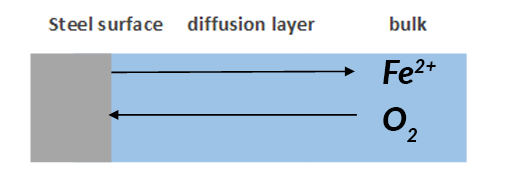NTIC is a “Critical Sector” company that remains open for business! NTIC Stakeholder Update On COVID-19 →

The corrosion rate is controlled by different factors such as pH, temperature, electrochemical potential, electrolyte, and as well as the applied corrosion control method. Those factors together make the corrosion control method evaluation complex and time-consuming.

As we all know, corrosion is an electrochemical procedure.  The concept of electrical resistance tells us the higher the electrical resistance, the lower current, and it leads to a lower corrosion rate.  If we treat the corrosive system as an electrochemical cell, measuring the corresponding current to the controlled potential, we are able to get the electrical resistance of the system. The overall electrical resistance is consist of the component such as resistors, capacitors, and inductors. If we have a good model to analyze the resistance, theoretically, we are able to distinguish which component of the resistance is the dominating element, as well as find out the root cause of system failure.Ohm’s law equation (1) only applies for simple circuit resistance, which does not depend on frequency or considers the phase shift for AC current. In order to obtain more information on circuit resistance (for components such as capacitor and inductor), which is called impedance for accuracy here, the applied excitation signal is AC potential with a range of frequency as well.

We can use the equivalent circuit model shown in Figure 1 and Figure 2 to analyze a metal with coating in corrosive conditions.  Where Rs is solution resistance, Rƒ is the coating film resistance; Cƒ is coating film capacitance; Rt is charge transfer resistance; Cdl is double layer capacitance; Zw Warburg impedance or diffusion impedance. Each of them is discussed later in this article.Figure 1: Equivalent circuit model for metal with coating.Figure 2: Equivalent circuit model for metal with coating. Coating resistance is described as pore resistance here.

Solution resistance or electrolyte resistance Rs is a significant factor in the impedance of an electrochemical cell. The resistance of the electrolyte solution depends on the ion types, concentration, temperature, and geometry of the electrode surface. For example, DI water has a much higher electrolyte resistance than a salt solution. Solution resistance is often read from the Nyquist Plot (the real axis value at the high-frequency intercept) in Figure 3.

Charge Transfer Resistance Rt, when a metal is in contact with an electrolyte, metal gets oxidized, so electrons leave the metal, and metal ions diffuse into the electrolyte. A charge is transferred during this process. The charge transfer resistance is greatly controlled by the reaction kinetics, the temperature, the concentration of the reactant and reaction products, and the potential. Sometimes, researchers will stir the solution to minimize the concentration gradient of the reactant/reaction product between the gradient between bulk and metal surface to study the charge-transfer kinetics.Diffusion or Warburg Impedance Zw is the impedance to evaluate species mass transfer from bulk to the metal surface. As mentioned earlier, there is always a concentration gradient between the bulk and metal surface. Therefore, the Warburg impedance highly depends on how fast the metal ion can be transferred from the metal surface to the bulk solution, and how fast the oxidant can be transferred from bulk to metal surface to consume the electrons (Figure 4). At the same time, Warburg impedance also depends on the frequency of the applied potential perturbation. Under low frequency, the reactants need to travel further for reaction, which increases the Warburg impedance. Vice versa under high frequency.Figure 4 diffusion layer caused by the reactant concentration gradient between bulk and metal surface.

Coating Capacitance Cƒ is typically used to evaluate the protection of the organic coating or hydrophobic layer formed on the surface. The capacitance depends on surface size, dielectric constants of the material, and distances between two plates, which is the coating thickness. At room temperature, the DI water has a dielectric constant of 80, while most of the organic coating has a dielectric constant below 10. The changed coating capacitance typically means the coating has absorbed water or has some defect generated. One typical defect is the generated pore in the coating, which provides extra paths for reactant and electron.

Double Layer Capacitance Cdl
A typical double layer on the surface of the steel is constructed by the adsorption of H₂O molecules at the metal/solution interface. The charged metal is separated from the charged ions by an insulating space function like a capacitance. This double layer is affected by open circuit electrode potential, temperature, ionic concentrations, types of ions, oxide layers, electrode roughness, and impurity adsorption. The existence of oxygen, and carbon dioxide will also push apart the double layer and cause a decrease in double-layer capacitance. The thickness of the double layer is in the order of angstroms. In the real cell, the double layer is typically in the double layer is not always an ideal capacitor, and it is always treated as an empirical constant.

References

BonnelA, & DabosiF. (1983). Corrosion Study of a Carbon Steel in Neutral Chloride Solutions by Impedance Techniques. Journal of the Electrochemical Society, 753-763.

Gamry. (n.d.). Basics of Electrochemical Impedance Spectroscopy. Retrieved from https://www.gamry.com/application-notes/EIS/basics-of-electrochemical-impedance-spectroscopy/

HongT, SunY.H, & JepsonW.P. (2002). Study on Corrosion Inhibitor in Larger Pipeline under Multiphase Flow using EIS. Corrosion Science, 101-112.

ZhengYougui. (2015). Electrochemical Mechanism and Model of H₂S Corrosion of Carbon Steel. Athens: Ohio University.